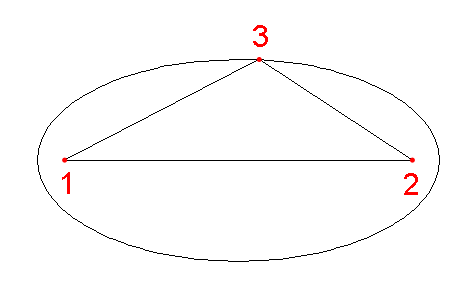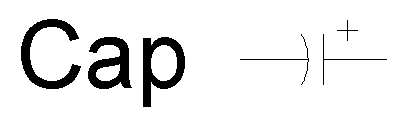Ellipse

Draw an ellipse using one of four methods.

Mode Settings:

Method Two Points | Center, A, B | Radius, Planar Rotation | Foci, Point Choose method

Two Points Method: Construct an ellipse from two points.

First Point Point First corner
Second Point Point Second corner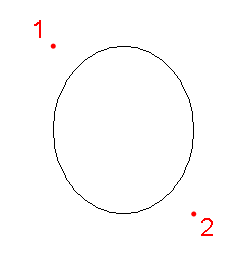Center, A, B Method: Construct an ellipse from a center, radius A, and radius B.

Center Point Center of the ellipse
Radius A Point or Distance > 0 Specify Radius A and rotation simultaneously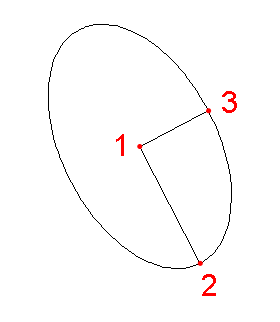Radius, Planar Rotation Method: Construct an ellipse from a radius and a planar rotation. A planar rotation of 0 creates a circle, while a planar rotation of 90 creates a line. This method simulates rotating a circle in 3D space with a top view.

Center Point Center of the ellipse
Radius A Point or Distance > 0 Specify Radius A and rotation simultaneously
Planar Rotation Point or Angle Planar rotation of the circle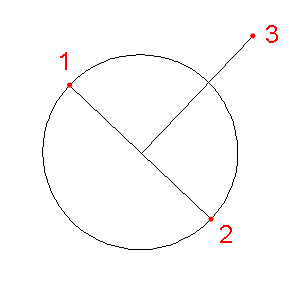Foci, Point Method: Construct an ellipse from two foci and a third point.